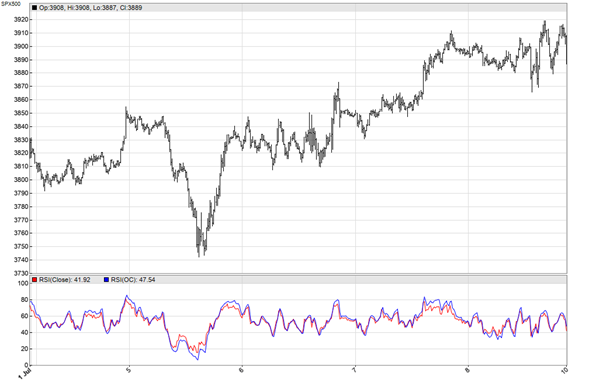# Open or Close? Why Not Both?In his TASC February 2023 article, John Ehlers proposed to use the average of open and close, rather than the close price, for technical indicators. The advantage is a certain amount of noise reduction. On intraday bars the open-close average is similar to an SMA(2). It makes the data a bit smoother, but at cost of additional lag by half a bar.

The script below, in C for the Zorro platform, compares the standard RSI with the open-close average RSI on the S&P 500 index with 15-minute bars:

```void run()
{
BarPeriod = 15;
StartDate = 20220629;
EndDate = 20220712;
asset("SPX500");
vars OC = series((priceO()+priceC())/2);
plot("RSI(Close)",RSI(seriesC(),14),NEW,RED);
plot("RSI(OC)",RSI(OC,14),0,BLUE);
}```

We can indeed see some noise reduction in the resulting chart:The obvious question: Will the smoother curve compensate for the additional lag in a trading system? For testing this, I added the following 5 lines to the script:

```vars RSIs = series(RSI(OC,14));
if(crossUnder(RSIs,70))
enterShort();
if(crossOver(RSIs,30))
enterLong();```

That’s a simple RSI trading system: Enter a short position when the RSI crosses below 70, and enter a long position when it crosses above 30. Any position is closed when an opposite position is opened. I found indeed that that using the open-close average produced a better result with some instruments and time periods, but a worse result with others. There was no clear tendency. However, it’s certainly worth a try when you’re anyway developing an indicator based trading system.

1.Arnis Lapsa says: